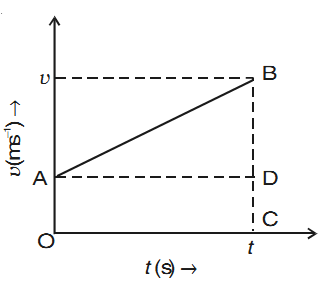# Second Equation of Uniformly Accelerated Motion

Second equation of motion is used to calculate the position of an object after time t when it is undergoing constant acceleration a.

Suppose that at t = 0, x1 = x0; v1 = v0 and at t = t, x2 = x; v2 = v.The distance traveled = area under v – t graph = Area of trapezium OABC

= ½(CB + OA) × OC

x – x0 = ½(v + v0)t

Since v = v0 + at,

x – x0 = ½(v0 + at + v0)t

x – x0 = v0t + ½at2

x = x0 + v0t + ½at2